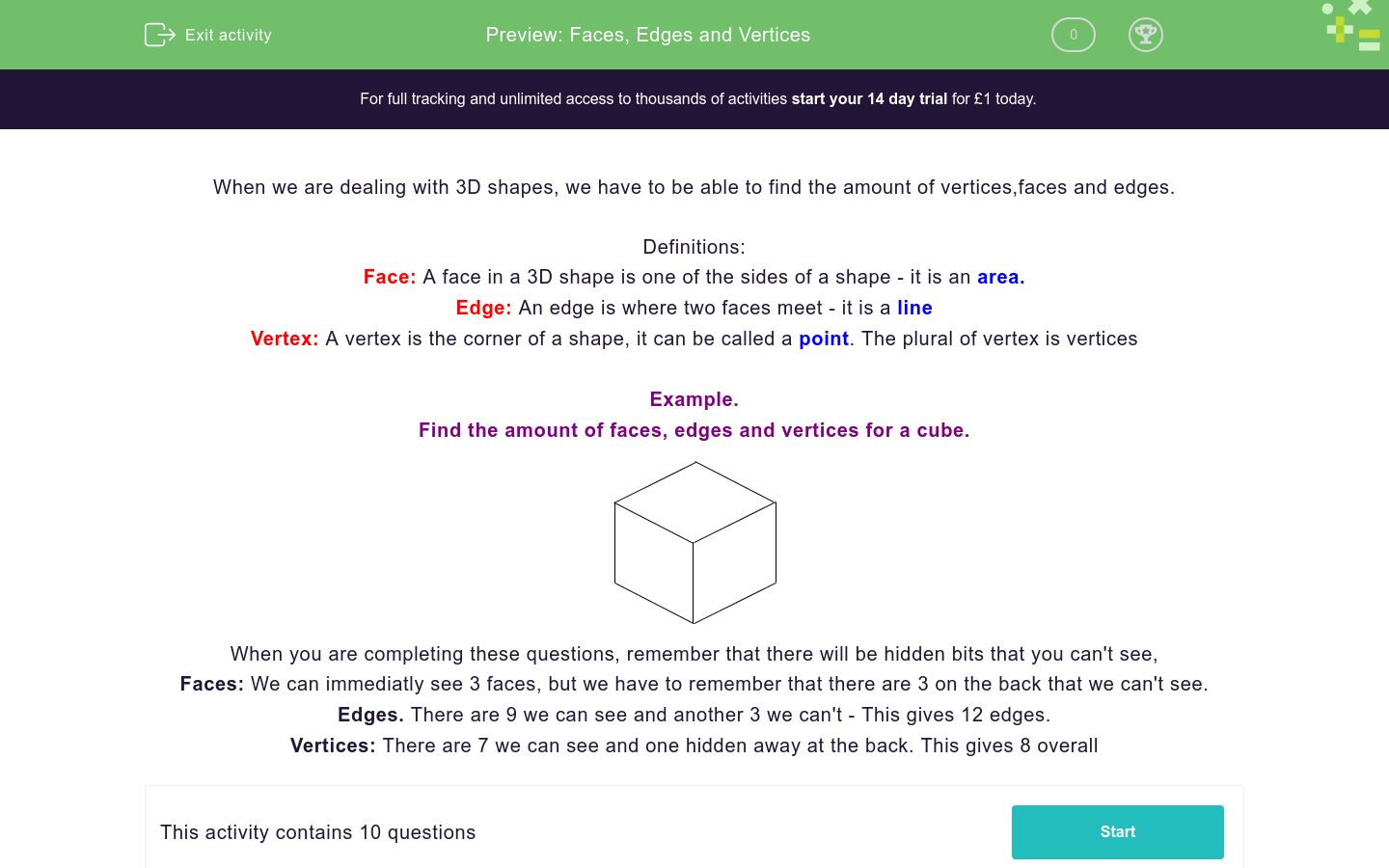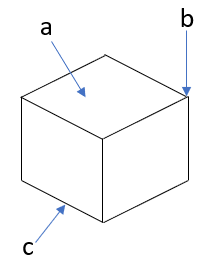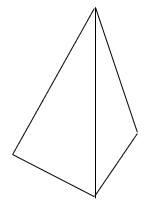# Faces, Edges and Vertices

In this worksheet, students practise finding the edges, faces and vertices for 3D shapes.Key stage:  KS 4

GCSE Subjects:   Maths

GCSE Boards:   AQA, Eduqas, Pearson Edexcel, OCR

Curriculum topic:   Geometry and Measures, Basic Geometry

Curriculum subtopic:   Properties and Constructions, Three-Dimensional Shapes

Difficulty level:### QUESTION 1 of 10

When we are dealing with 3D shapes, we have to be able to find the amount of vertices,faces and edges.

Definitions:

Face: A face in a 3D shape is one of the sides of a shape - it is an area.

Edge: An edge is where two faces meet - it is a line

Vertex: A vertex is the corner of a shape, it can be called a point. The plural of vertex is vertices

Example.

Find the amount of faces, edges and vertices for a cube.When you are completing these questions, remember that there will be hidden bits that you can't see,

Faces: We can immediatly see 3 faces, but we have to remember that there are 3 on the back that we can't see.

Edges. There are 9 we can see and another 3 we can't - This gives 12 edges.

Vertices: There are 7 we can see and one hidden away at the back. This gives 8 overall

Match the letter with the feature of a 3D shape.## Column B

A
face
B
edge
C
Vertex

Complete the definitions...

## Column B

A
face
B
edge
C
Vertex

How many vertices does a cuboid have?

How many faces does a cuboid have?

How many edges does a cuboid have?

Order these shapes by the number of faces.

Put 1 next to the shape with the most faces, 2 next to the one with the next highest amount of faces etc.

 Order Cuboid Triangular prism hexagonal prism triangular based pyramid

Order these shapes by the number of vertices.

Put 1 next to the shape with the most faces, 2 next to the one with the next highest amount of faces etc.

 Order Cuboid Triangular prism pentagonal prism triangular based pyramid

A tetrehedron has...Order Cuboid Triangular prism pentagonal prism triangular based pyramid

The prism shown here has...Order Cuboid Triangular prism pentagonal prism triangular based pyramid

A sphere has

 Order Cuboid Triangular prism pentagonal prism triangular based pyramid
• Question 1

Match the letter with the feature of a 3D shape.## Column B

A
face
B
Vertex
C
edge
EDDIE SAYS
Remember the rules. Face -> Area Edge -> Line Vertex -> Point
• Question 2

Complete the definitions...

EDDIE SAYS
Unfortunately there isn't a quick way to remember these, you just have to memorise them.
• Question 3

How many vertices does a cuboid have?

8
EDDIE SAYS
Remember that vertices are the pointy bits! How many are there on a cuboid?
• Question 4

How many faces does a cuboid have?

6
EDDIE SAYS
Remember that vertices are the flat bits with an area How many are there on a cuboid?
• Question 5

How many edges does a cuboid have?

12
EDDIE SAYS
Remember that edges are the straight lines. How many do we need to draw all the lines for a cuboid?
• Question 6

Order these shapes by the number of faces.

Put 1 next to the shape with the most faces, 2 next to the one with the next highest amount of faces etc.

 Order Cuboid Triangular prism hexagonal prism triangular based pyramid
EDDIE SAYS
With these, you need to know how many faces each one has. A cuboid has 6 A triangular prism has 5 A hexagonal prism has 8 A triangular based pyramid has 4 Once you have this, you can order them quite easily
• Question 7

Order these shapes by the number of vertices.

Put 1 next to the shape with the most faces, 2 next to the one with the next highest amount of faces etc.

 Order Cuboid Triangular prism pentagonal prism triangular based pyramid
EDDIE SAYS
With these, you need to know how many faces each one has. A cuboid has 12 A triangular prism has 6 A pentagonal prism has 10 A triangular based pyramid has 4 Once you have this, you can order them quite easily
• Question 8

A tetrehedron has...EDDIE SAYS
A tetrahedron is just a triangular based pyramid where all the faces are the same. Don\'t forget to include the things you can\'t see on the picture.
• Question 9

The prism shown here has...EDDIE SAYS
This is a bit more challenging but it's still the same process. There will be one hidden face on the far side, one on the back and one on the bottom. For the edges, there are 6 on the end so there must be 6 on the other end and one hidden at the back
• Question 10

A sphere has

EDDIE SAYS
Think logically about this. How many points does a sphere have? How many lines does a sphere have?
---- OR ----

Sign up for a £1 trial so you can track and measure your child's progress on this activity.

### What is EdPlace?

We're your National Curriculum aligned online education content provider helping each child succeed in English, maths and science from year 1 to GCSE. With an EdPlace account you’ll be able to track and measure progress, helping each child achieve their best. We build confidence and attainment by personalising each child’s learning at a level that suits them.

Get started